Analytics

# Uncubing Data: Using SQL to Expand Aggregated Data and Prevent Data Loss

By Charles Zhu - December 15, 2020

Any time you aggregate data, you lose the information contained at the finer grain. At Sisu, we’re constantly applying new techniques to prevent that loss of information for our customers because we know with more features, an analysis can be more comprehensive and accurate.

While wide, flat data is the ideal, sometimes you might only have access to aggregated data, like Google or Facebook Ads reports. These tools export summary data where the primary key is utm_campaign and day (like in the table below), instead of granularity at the level of a click or even a lead.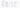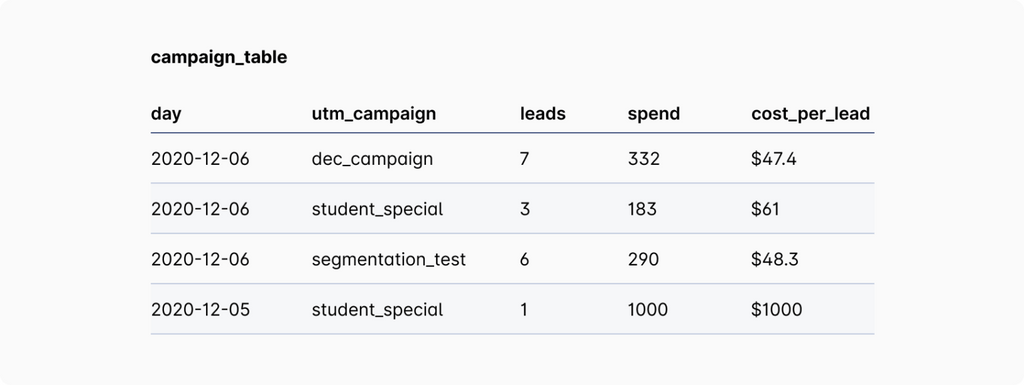These reports have to provide data in the aggregate because granular data are too difficult for most users to process. As is, this type of table is ready-made for visualizing the daily performance of metrics, but doesn’t leave much room for more in-depth analysis. You can only aggregate upwards to, for example, a Cost Per Lead (CPL) per day metric by summing up the spend across a day and then dividing it by the number of leads:

In: SELECT day, sum(leads) AS total_leads, sum(spend) AS total_spend, (sum(spend) / sum(leads)) AS blended_cpl FROM campaign_table GROUP BY day 
Out: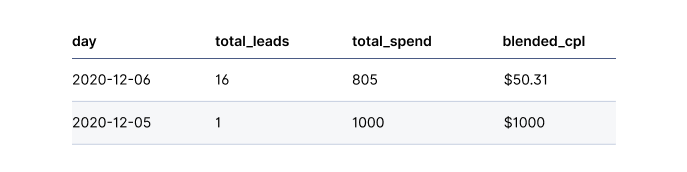But if we need to quickly get average CPL across many different subpopulations across different factor values, requiring GROUP BYs can get tedious. Ideally, we can use AVG to aggregate rows and the WHERE clause to select for any subpopulation.

## Disaggregate tables to simplify queries and find accurate insights

To elaborate, let’s imagine you want to know the average CPL for the student_special campaign in the original table. If you used the query:

SELECT AVG(cost_per_lead) FROM campaign_table WHERE utm_campaign = ‘student_special’

you would generate an incorrect answer of $530.50. The true answer is ($183 + $1000) / 4 or$295.75 because the number of leads is different on 2020-12-05 vs. 2020-12-06. The CPL metric needs to be weighted appropriately by the number of leads.

The correct approach is to GROUP BY utm_campaign and derive average CPL by dividing SUM(spend) by SUM(leads). But if I want to select any different average CPLs across many different subpopulations across different factor values, the query quickly grows in complexity.

Instead, we can model the data at a different grain and disaggregate the table by making each row equivalent to one lead. This approach would produce a table similar to the one below: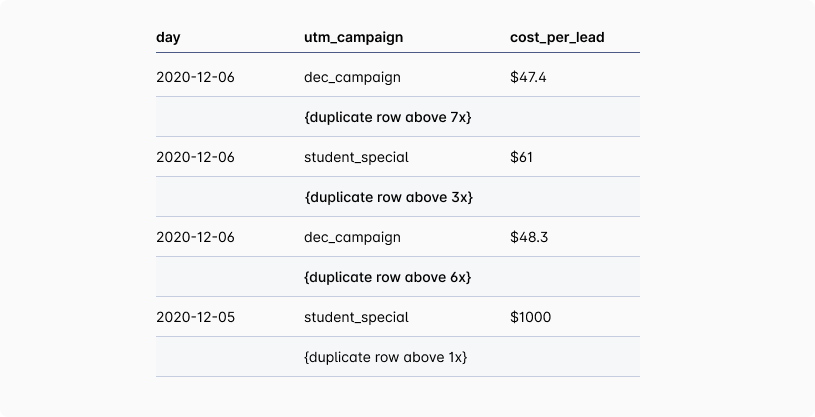This table enables simple averages of cost_per_lead, without having to GROUP BY and then use SUM(spend) / SUM(leads). Now, if we were to use the query above again on this new table,

SELECT AVG(cost_per_lead) FROM campaign_table WHERE utm_campaign = 'student_special'

it will return the accurate CPL and replaces the need to use GROUP BY. While generating this table with a new row for each lead does take up much more space, the shift to cloud data warehousing can make the additional costs marginal.

## A SQL approach to disaggregating

While breaking each lead out by row is simple in Python using a simple row multiplication command, doing the same thing in SQL is more complicated. Here’s one approach to solve this in SQL:

With ten_numbers AS (SELECT 1 AS num UNION SELECT 2 UNION SELECT 3 UNION SELECT 4 UNION SELECT 5 UNION SELECT 6 UNION SELECT 7 UNION SELECT 8 UNION SELECT 9 UNION SELECT 0), gen_numbers AS ( SELECT (10000 * t1.num) + (1000 * t2.num) + (100 * t3.num) + (10 * t4.num) + t5.num AS gen_num FROM ten_numbers AS t1 JOIN ten_numbers AS t2 ON 1 = 1 JOIN ten_numbers AS t3 ON 1 = 1 JOIN ten_numbers AS t4 ON 1 = 1 JOIN ten_numbers AS t5 ON 1 = 1 WHERE (10000 * t1.num) + (1000 * t2.num) + (100 * t3.num) + (10 * t4.num) + t5.num > 0 ORDER BY gen_num ) 

Let’s break down this query. The first two CTEs, ten_numbers and gen_numbers, create a single-column table listing numbers from 0 to 99999. Ten_numbers is a list of ten numbers. Then, by joining it to itself several times, we expand the ten number list so that each set of ten numbers occurs in a different place holder in the number:

• The “WHERE 1=1” clause creates all possible combinations of the initial vector of ten numbers with the same ten numbers in a different column, i.e. [0,0]; [0,1]…[0,10]; [1,0]; [1,1]…[10,10]
• The “SELECT” statement at the top ensures that each combination takes place at a different placeholder in the number, e.g., the ones digit, the tens digit, the hundreds digit, etc.

With this matrix of numbers in place, we can now join our leads table with the vector of numbers we just created:

SELECT campaign_table.* FROM campaign_table AS t JOIN gen_numbers AS g ON 1 = 1 WHERE g.gen_num <= t.leads

By again joining on 1=1, we create every possible combination of rows in the campaign_table with each number in the gen_numbers table, automatically duplicating each campaign_table row 99,999 times. Then, by using the WHERE g.gen_num <= t.leads, we downsize the number of duplicates to correspond to the number of leads in the original table.

## Using scaled weights to deal with gigantic tables

This method of disaggregating tables is memory intensive and will become less worthwhile if rows need to be duplicated millions of times. But we can easily make this type of weighting accurate to a certain percentage point by abstracting the number of leads and creating a simple weights column.

For example, in the table below, you don’t need to create 17 million rows.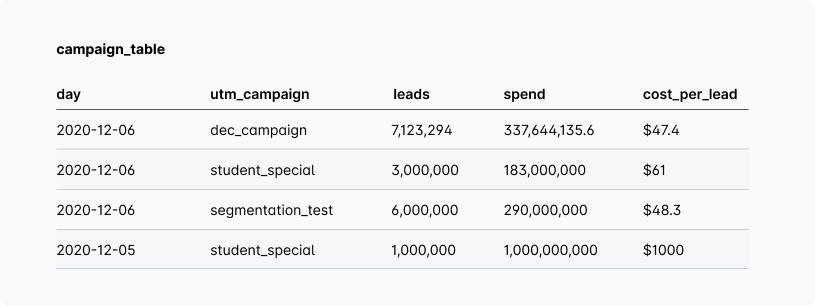Instead, you can create a new column that divides the cost_per_leads value by the sum of leads and specify a floating point decimal – this gets you the proportion of total leads that this particular group contributed.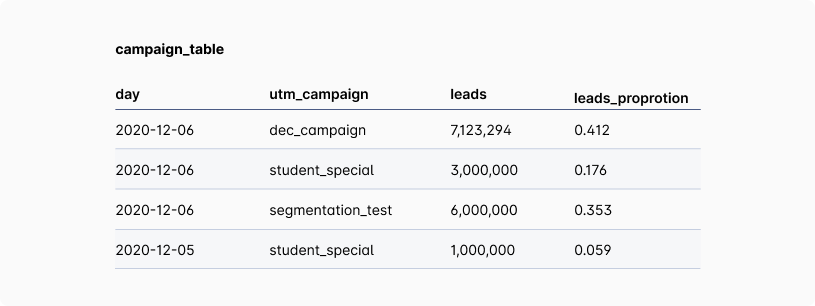Then, multiply the leads proportion column by 100 or 1,000 to get to a 1% or a 0.1% accuracy, as needed. As a result, the gen_numbers table can be scaled down from 99,999 initial duplicates to 99 for 1% accuracy or 999 for 0.1% accuracy.

gen_numbers AS ( SELECT (100 * t3.num) + (10 * t4.num) + t5.num AS gen_num FROM ten_numbers AS t3 ON 1 = 1 JOIN ten_numbers AS t4 ON 1 = 1 JOIN ten_numbers AS t5 ON 1 = 1 WHERE (100 * t3.num) + (10 * t4.num) + t5.num > 0 ORDER BY gen_num )

Now, you are limited to duplicating each row only 999 times in the initial self join.

With an aggregate table that’s been artificially disaggregated to a lower grain, it becomes quicker and easier to account for a weight, like the number of leads or the amount of spend. Now, we can get averages across multiple subpopulations with a simple WHERE clause, making a scan across different factors, columns, and date periods much easier. This dramatically expands the size of the table, but with cheap storage and lightning-fast compute, it’s a trade-off that could be worth making.

Looking to do more with your granular and aggregate data? View our Designing Better Datasets guide or get in touch with our team for help analyzing your key metrics.